Next: Electric Potential and Electric Up: Electric Potential Previous: Electric Potential Energy

## Electric Potential

Consider a chargeplaced in an electric field generated by fixed charges. Let us chose some arbitrary reference pointin the field. At this point, the electric potential energy of the charge is defined to be zero. This uniquely specifies the electric potential energy of the charge at every other point in the field. For instance, the electric potential energy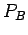at some pointis simply the work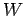done in moving the charge fromtoalong any path. Now,can be calculated using Eq. (78). It is clear, from this equation, thatdepends both on the particular chargewhich we place in the field, and the magnitude and direction of the electric field along the chosen route between pointsand. However, it is also clear thatis directly proportional to the magnitude of the charge. Thus, if the electric potential energy of a chargeat pointisthen the electric potential energy of a charge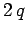at the same point is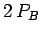. We can exploit this fact to define a quantity known as the electric potential. The difference in electric potential between two pointsandin an electric field is simply the work done in moving some charge between the two points divided by the magnitude of the charge. Thus,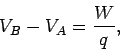(80)

where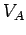denotes the electric potential at point, etc. This definition uniquely defines the difference in electric potential between pointsand, but the absolute value of the potential at pointremains arbitrary. We can therefore, without loss of generality, set the potential at pointequal to zero. It follows that the potential energy of a chargeat some pointis simply the product of the magnitude of the charge and the electric potential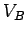at that point: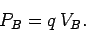(81)

It is clear, from a comparison of Eqs. (78) and (79), that the electric potential at point(relative to point) is solely a property of the electric field, and is, therefore, the same for any charge placed at that point. We shall see exactly how the electric potential is related to the electric field later on.

The dimensions of electric potential are work (or energy) per unit charge. The units of electric potential are, therefore, joules per coulomb (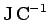). A joule per coulomb is usually referred to as a volt (V): i.e.,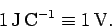(82)

Thus, the alternative (and more conventional) units of electric potential are volts. The difference in electric potential between two points in an electric field is usually referred to as the potential difference, or even the difference in voltage,'' between the two points.

A battery is a convenient tool for generating a difference in electric potential between two points in space. For instance, a twelve volt (12V) battery generates an electric field, usually via some chemical process, which is such that the potential difference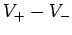between its positive and negative terminals is twelve volts. This means that in order to move a positive charge of 1 coulomb from the negative to the positive terminal of the battery we must do 12 joules of work against the electric field. (This is true irrespective of the route taken between the two terminals). This implies that the electric field must be directed predominately from the positive to the negative terminal.

More generally, in order to move a chargethrough a potential difference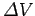we must do work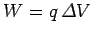, and the electric potential energy of the charge increases by an amount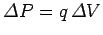in the process. Thus, if we move an electron, for which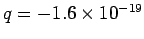C, through a potential difference of minus 1 volt then we must do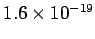joules of work. This amount of work (or energy) is called an electronvolt (eV): i.e.,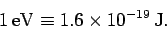(83)

The electronvolt is a convenient measure of energy in atomic physics. For instance, the energy required to break up a hydrogen atom into a free electron and a free proton is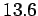eV.Next: Electric Potential and Electric Up: Electric Potential Previous: Electric Potential Energy
Richard Fitzpatrick 2007-07-14